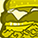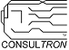# PSoC™ Creator & Designer Forum DiscussionsLevel 2
Level 2# Defining an Array of constants

Hi,

I have a program with PSOC5 where I have a multichannel ADC. I want to be able to configure the zero error and gain of each channel individually so that I can use the below code.

------------------------------

And have a definition such that

Zero_Error=100;

Zero_Error=30;

Zero_Error=-70;

.... and so on.

I have been simply using variable arrays to store Zero_Error and Gain_x100 but I am sure that there is a better way to do this.

Obviously I can't use #define.

1 SolutionLevel 9
Level 9# Re: Defining an Array of constants

sujoy,

If you already know the 'constants' for each channel, you should use the following lines

``````#define No_of_ADC_Channels  16 //  Enter the # of channels here
const uint16 Zero_Error[No_of_ADC_Channels] = {100, 30, -70, ..., 128};
const uint16 Gain_x100[No_of_ADC_Channels] = {1, 1, 2, ..., 4};
``````

The 'const' in the line defines that the array is FLASH.   Therefore the memory is only changeable at design-time.

A more unified method of coding is to incorporate both variables in a struct array.  Example:

``````#define No_of_ADC_Channels  16 //  Enter the # of channels here
/* Here is the struct definition that combines the two correction factors */
typedef struct
{
const uint16 Gain_x100;
const uint16 Zero_Error;
} gain_offset_s;

{ /* Gain_x100, Zero_Error */
{          1, 100 },
{          1, 30},
{          2, -70},
...
{          4, 128},
};

/* Example use in a function */
function()
{
uint16 gain;
uint16 zero;
...
...
}``````

If you need to calibrate each channel for every unit, then you need to at least store the data in RAM.   In this case, you should take the code lines above and remove the 'const'.   It will place the arrays in SRAM.  You can even use the = {...} values to be default values.

I assume you have functions that can executed to calibrate the gain and offset of each channel.

Once you have SRAM array variables as you currently allocate, you may want to use the PSoC5 EEPROM to store these arrays.   That way if you lose power or get a watchdog reset, the offset and gain values can be reloaded into the SRAM arrays.

Len
"Engineering is an Art. The Art of Compromise."Level 9
Level 9# Re: Defining an Array of constants

sujoy,

If you already know the 'constants' for each channel, you should use the following lines

``````#define No_of_ADC_Channels  16 //  Enter the # of channels here
const uint16 Zero_Error[No_of_ADC_Channels] = {100, 30, -70, ..., 128};
const uint16 Gain_x100[No_of_ADC_Channels] = {1, 1, 2, ..., 4};
``````

The 'const' in the line defines that the array is FLASH.   Therefore the memory is only changeable at design-time.

A more unified method of coding is to incorporate both variables in a struct array.  Example:

``````#define No_of_ADC_Channels  16 //  Enter the # of channels here
/* Here is the struct definition that combines the two correction factors */
typedef struct
{
const uint16 Gain_x100;
const uint16 Zero_Error;
} gain_offset_s;

{ /* Gain_x100, Zero_Error */
{          1, 100 },
{          1, 30},
{          2, -70},
...
{          4, 128},
};

/* Example use in a function */
function()
{
uint16 gain;
uint16 zero;
...